# System of Linear Equations SCVASU 1 Linear Equations

• Slides: 13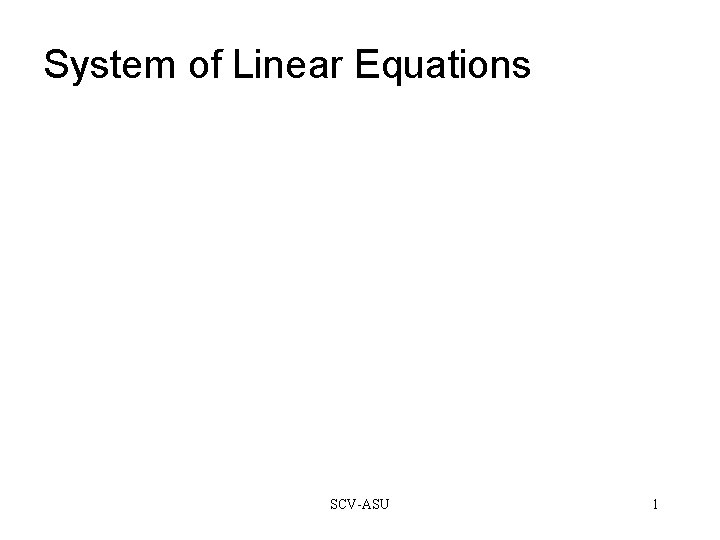System of Linear Equations SCV-ASU 1Linear Equations A system of linear equations is usually referred to as: Ax = b Where A is the matrix of the coefficients, x is a one dimensional matrix of unknowns, and b is a one dimensional matrix of answers. Here is an example: This system has two linear equations. This system in matrix form looks like this: So what are the sizes of these three matrices? SCV-ASU 2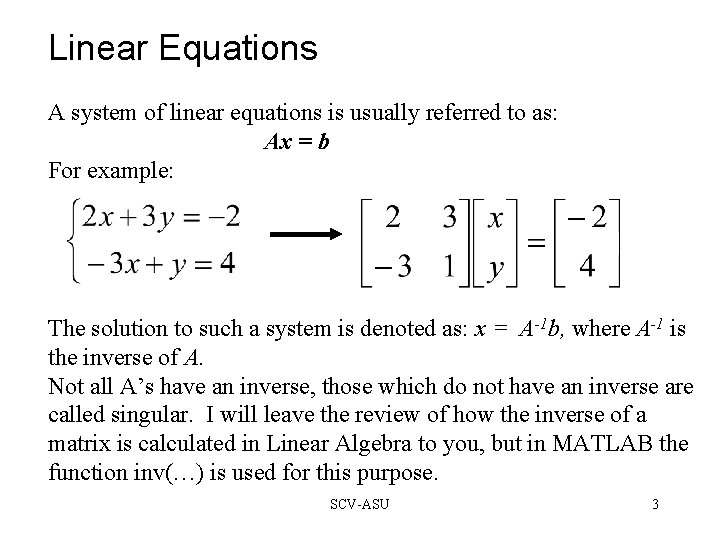Linear Equations A system of linear equations is usually referred to as: Ax = b For example: The solution to such a system is denoted as: x = A-1 b, where A-1 is the inverse of A. Not all A’s have an inverse, those which do not have an inverse are called singular. I will leave the review of how the inverse of a matrix is calculated in Linear Algebra to you, but in MATLAB the function inv(…) is used for this purpose. SCV-ASU 3Solution to Linear Equations So how can we solve this problem? One quick way is to include the unity matrix on the right-hand-side of the coefficient matrix as shown below and go through the process to bring that unity matrix to the left. Apply the operation, whatever it is, on the right-hand-side of the above equation. We will turn this into 1 This is into 0 This into 1 This into 0 SCV-ASU 4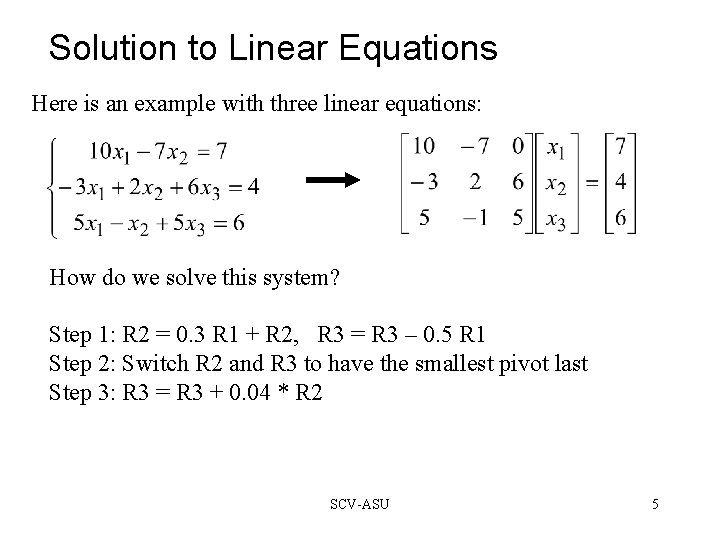Solution to Linear Equations Here is an example with three linear equations: How do we solve this system? Step 1: R 2 = 0. 3 R 1 + R 2, R 3 = R 3 – 0. 5 R 1 Step 2: Switch R 2 and R 3 to have the smallest pivot last Step 3: R 3 = R 3 + 0. 04 * R 2 SCV-ASU 5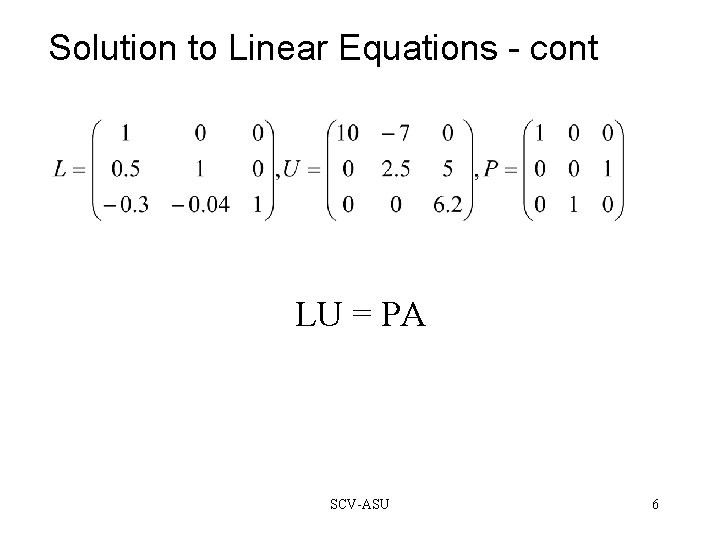Solution to Linear Equations - cont LU = PA SCV-ASU 6LU Decomposition This one of the oldest numerical techniques. Gaussian elimination has two stages: forward elimination and back substitution. This involves n-1 steps. At the kth step, multiples of the kth equation are subtracted from the remaining equations to eliminate the kth variable. If the coefficient of xk is “small” switching equations (pivoting) is recommended. SCV-ASU 7LU Decomposition - cont The process in summary: U = Mn-1 Pn-1 … M 2 P 2 M 1 P 1 A This can be written as: L 1 L 2 … Ln-1 U = Pn-1 …P 2 P 1 A Where Lk is obtained from Mk by permuting and changing the signs of the multipliers below the diagonal. Compact definition: L = L 1 L 2 … Ln-1 P = Pn-1 …P 2 P 1 LU = PA Let’s go back now to our example. SCV-ASU 8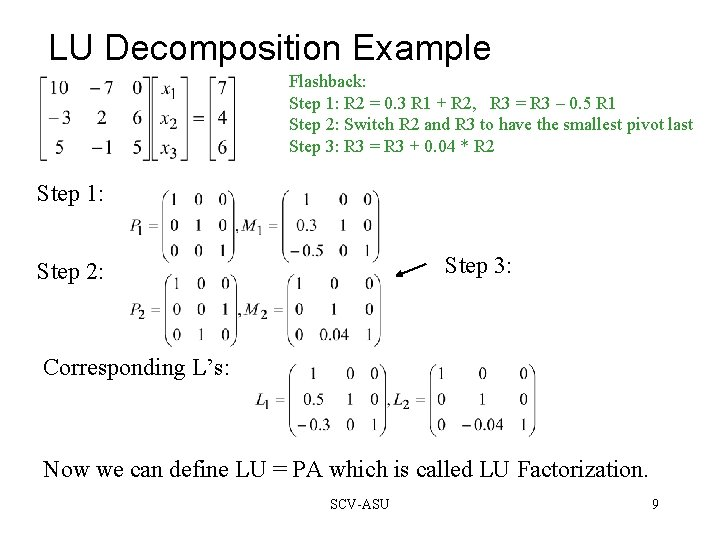LU Decomposition Example Flashback: Step 1: R 2 = 0. 3 R 1 + R 2, R 3 = R 3 – 0. 5 R 1 Step 2: Switch R 2 and R 3 to have the smallest pivot last Step 3: R 3 = R 3 + 0. 04 * R 2 Step 1: Step 3: Step 2: Corresponding L’s: Now we can define LU = PA which is called LU Factorization. SCV-ASU 9Why Pivoting Let’s look at the same example with a small change: Let’s solve this with 4 significant digits. SCV-ASU 10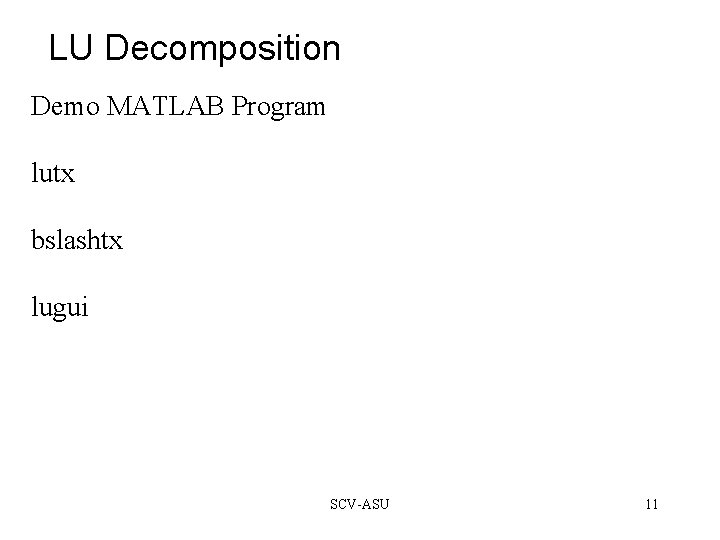LU Decomposition Demo MATLAB Program lutx bslashtx lugui SCV-ASU 11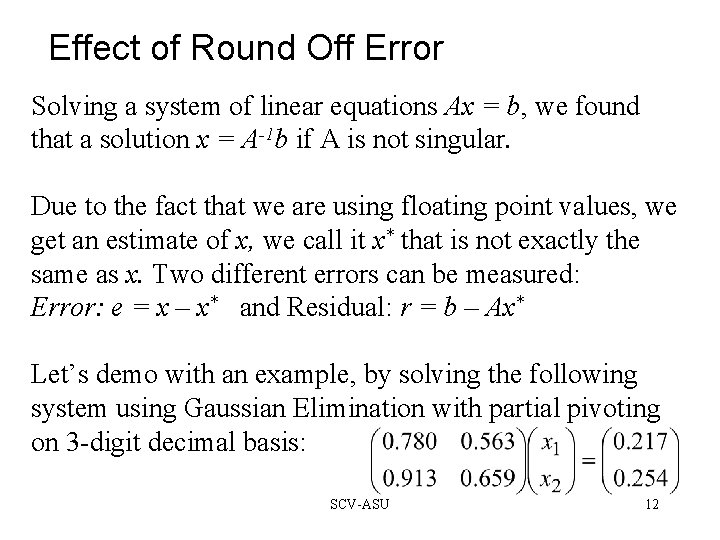Effect of Round Off Error Solving a system of linear equations Ax = b, we found that a solution x = A-1 b if A is not singular. Due to the fact that we are using floating point values, we get an estimate of x, we call it x* that is not exactly the same as x. Two different errors can be measured: Error: e = x – x* and Residual: r = b – Ax* Let’s demo with an example, by solving the following system using Gaussian Elimination with partial pivoting on 3 -digit decimal basis: SCV-ASU 12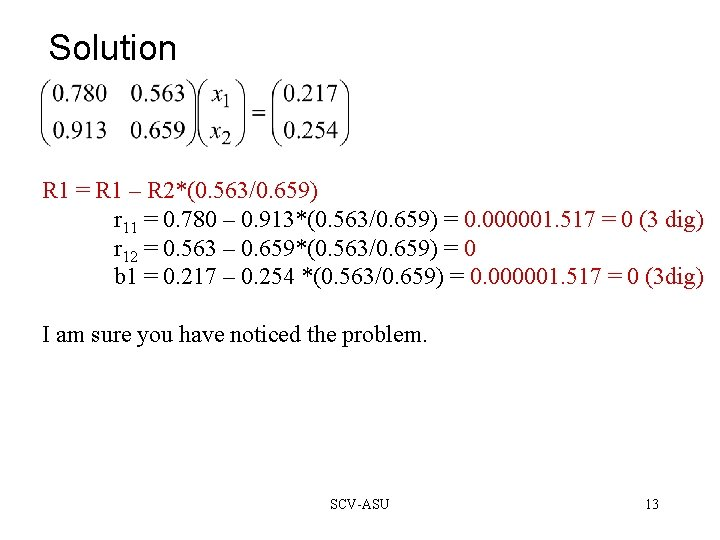Solution R 1 = R 1 – R 2*(0. 563/0. 659) r 11 = 0. 780 – 0. 913*(0. 563/0. 659) = 0. 000001. 517 = 0 (3 dig) r 12 = 0. 563 – 0. 659*(0. 563/0. 659) = 0 b 1 = 0. 217 – 0. 254 *(0. 563/0. 659) = 0. 000001. 517 = 0 (3 dig) I am sure you have noticed the problem. SCV-ASU 13